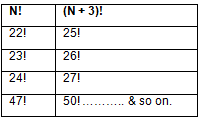Courses

# N! is ending with x zeroes. (N + 3)! is ending with (x + 2) zeroes. How many such N's will exist, given N is a two-digit number?a)5b)12c)10d)8Correct answer is option 'B'. Can you explain this answer? Related Test: CAT Mock Test - 1 (New Pattern)

## CAT Question

By Akanksha Gusain · 3 days ago ·CAT
Since we need 2 zeroes extra ⇒ (n + 3)! Should contain a multiple of 25.
So,So, There will exist 3 values of N corresponding to every multiple of 25.
So, N = 22, 23, 24, 47, 48, 49, 72, 73, 74, 97, 98, 99